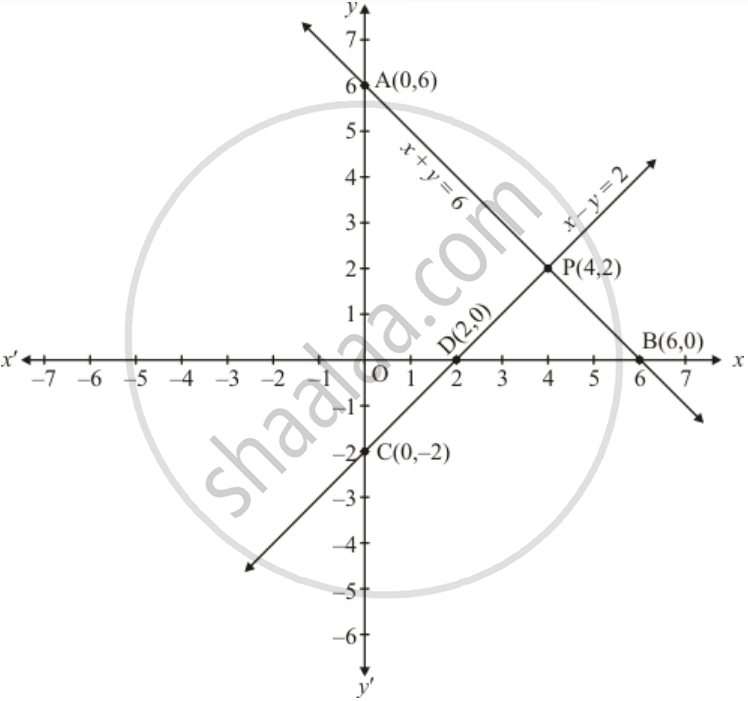Share

# Solve the Following Systems of Equations Graphically: X + Y = 6 X − Y = 2 - CBSE Class 10 - Mathematics

ConceptGraphical Method of Solution of a Pair of Linear Equations

#### Question

Solve the following systems of equations graphically:

x + y = 6

x − y = 2

#### Solution

The given equations are

x + y = 6 ...(1)

x − y = 2 ...(2)

Putting x = 0 in equation (1)we get

=> 0 + y = 6

=> y = 6

x = 0, y = 6

Putting y = 0 in equation (1) we get

=> x + 0 = 6

=> x = 6

Use the following table to draw the graph.

 x 0 6 y 6 0

Draw the graph by plotting the two points A(0,6) and B(6,0) from the tableGraph of the equation   ... (2)

x - y = 2 ..(2)

Putting x = 0 in equation (2) we get

=> 0 - y = 2

=> y = -2

x = 0, y = -2

Putting y = 0 in equation (2) we get

=> x - 0 = 2

=> x = 2

x = , y = 0

Use the following table to draw the graph.

 x 0 2 y -2 0

Draw the graph by plotting the two points C(0,-2) and D(2,0) from table.

The two lines intersect at points P(4,2).

Hence x = 4, y = 2 is the solution.

Is there an error in this question or solution?

#### APPEARS IN

Solution Solve the Following Systems of Equations Graphically: X + Y = 6 X − Y = 2 Concept: Graphical Method of Solution of a Pair of Linear Equations.
S